|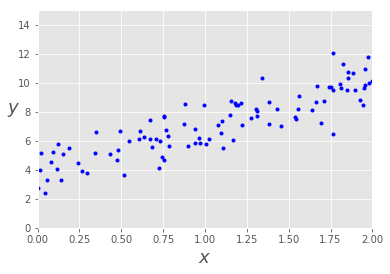y=b+mx

$$y=b+mx$$

J(θ)=θ0+θ1x

$$J(θ)=θ0+θ1x$$

|

|

|

|

|

|

Cost

J(θ)=1/2mm∑i=1(h(θ)(i)−y(i))2

$$J(θ)=1/2m∑i=1m(h(θ)(i)−y(i))2$$

∂J(θ)∂θj=1/mm∑i=1(h(θ(i)−y(i)).X(i)j

$$∂J(θ)∂θj=1/m∑i=1m(h(θ(i)−y(i)).Xj(i)$$

θ0:=θ0−α.(1/m.m∑i=1(h(θ(i)−y(i)).X(i)0)

$$θ0:=θ0−α.(1/m.∑i=1m(h(θ(i)−y(i)).X0(i))$$

θ1:=θ1−α.(1/m.m∑i=1(h(θ(i)−y(i)).X(i)1)

$$θ1:=θ1−α.(1/m.∑i=1m(h(θ(i)−y(i)).X1(i))$$

θ2:=θ2−α.(1/m.m∑i=1(h(θ(i)−y(i)).X(i)2)

$$θ2:=θ2−α.(1/m.∑i=1m(h(θ(i)−y(i)).X2(i))$$

θj:=θj−α.(1/m.m∑i=1(h(θ(i)−y(i)).X(i)0)

$$θj:=θj−α.(1/m.∑i=1m(h(θ(i)−y(i)).X0(i))$$

|

|

|
|

|

|
|

|

|

|

|

|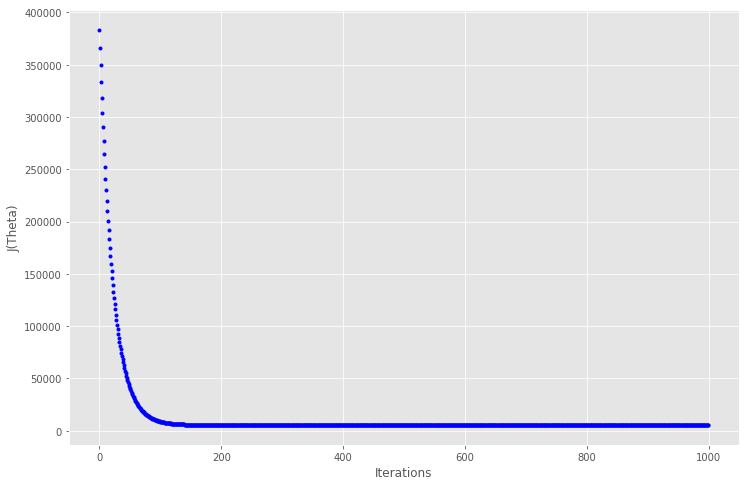|

|

|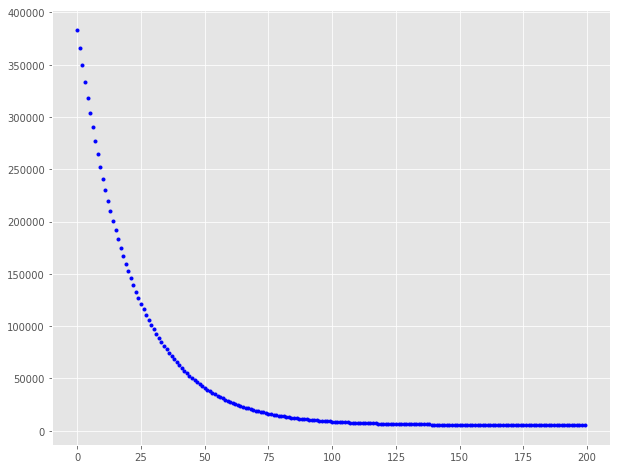|

|

|
|

|

|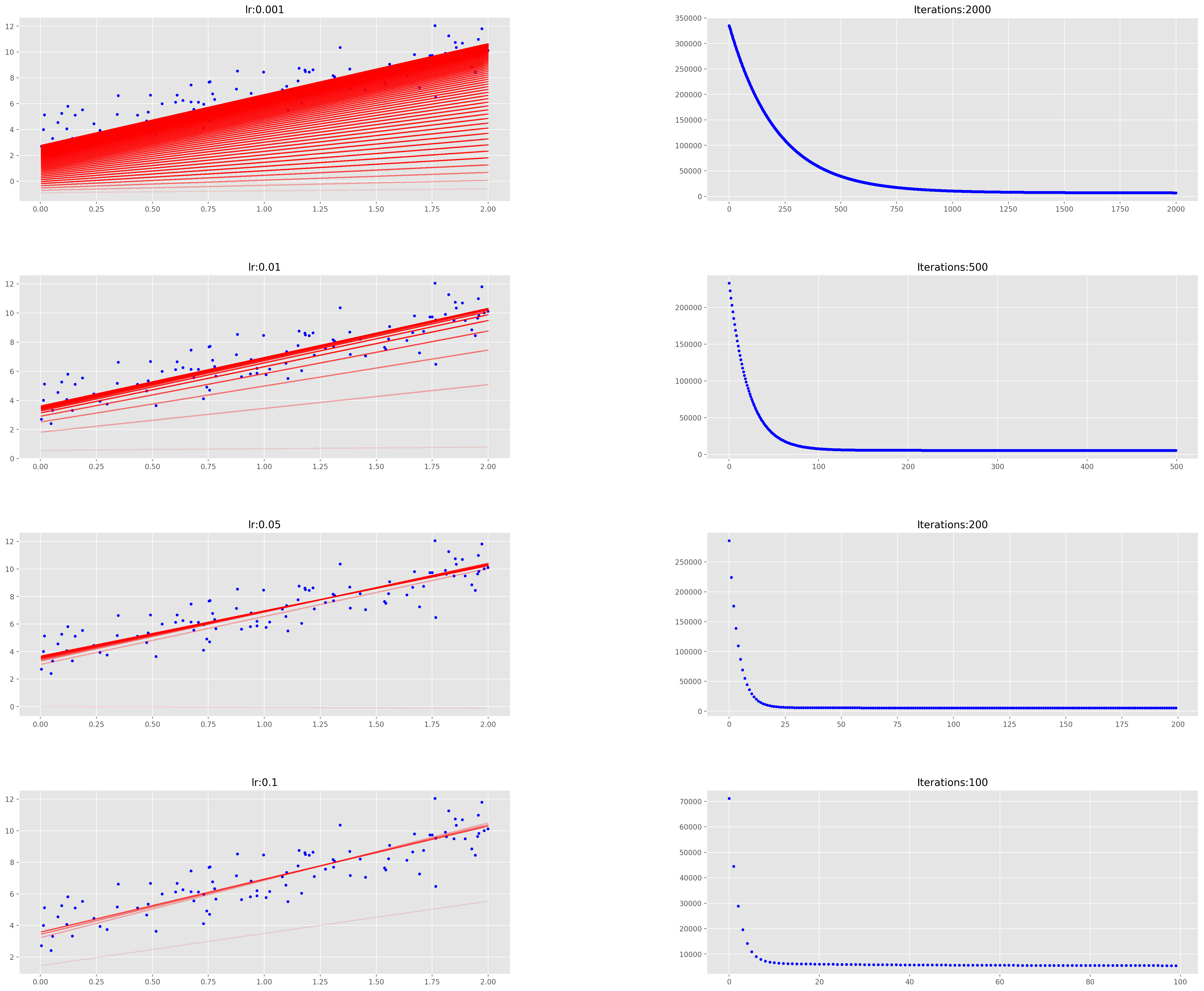|

|

|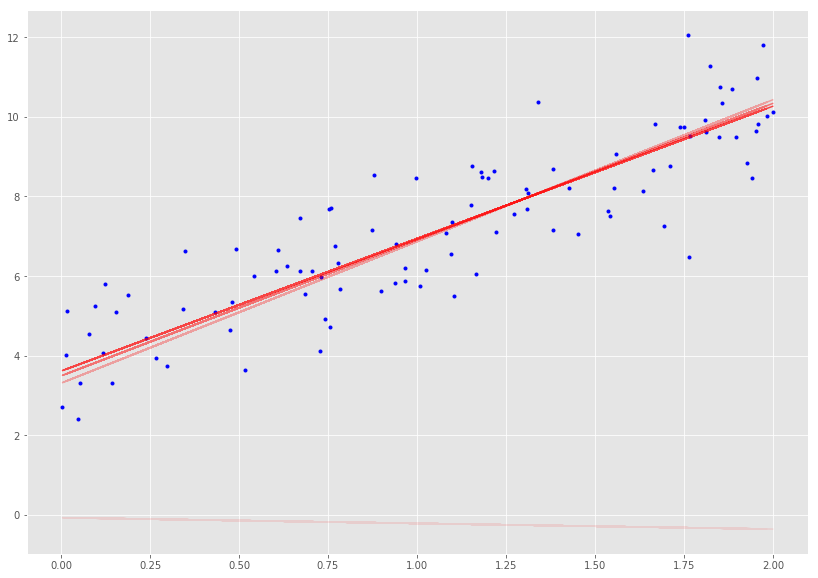θi=θi−α(hθ(x(j)0,x(j)1,…x(j)n)−yj)x(j)i

$$θi=θi−α(hθ(x0(j),x1(j),…xn(j))−yj)xi(j)$$

|

|

|
|

|

|

|

|

|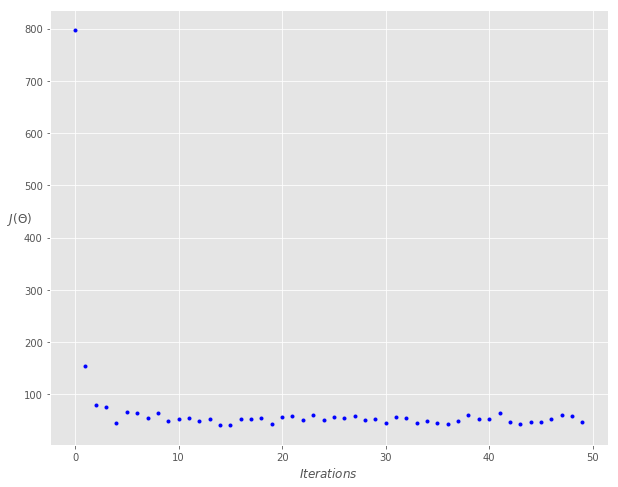θi=θi−αt+x−1∑j=t(hθ(x(j)0,x(j)1,…x(j)n)−yj)x(j)i

$$θi=θi−α∑j=tt+x−1(hθ(x0(j),x1(j),…xn(j))−yj)xi(j)$$

|

|

|
|

|

|

|

|

|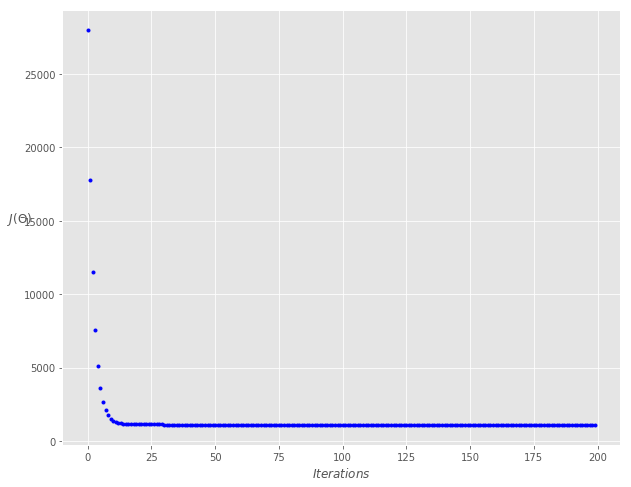_参考_：

laugh12321

2019-03-09

2020-10-23